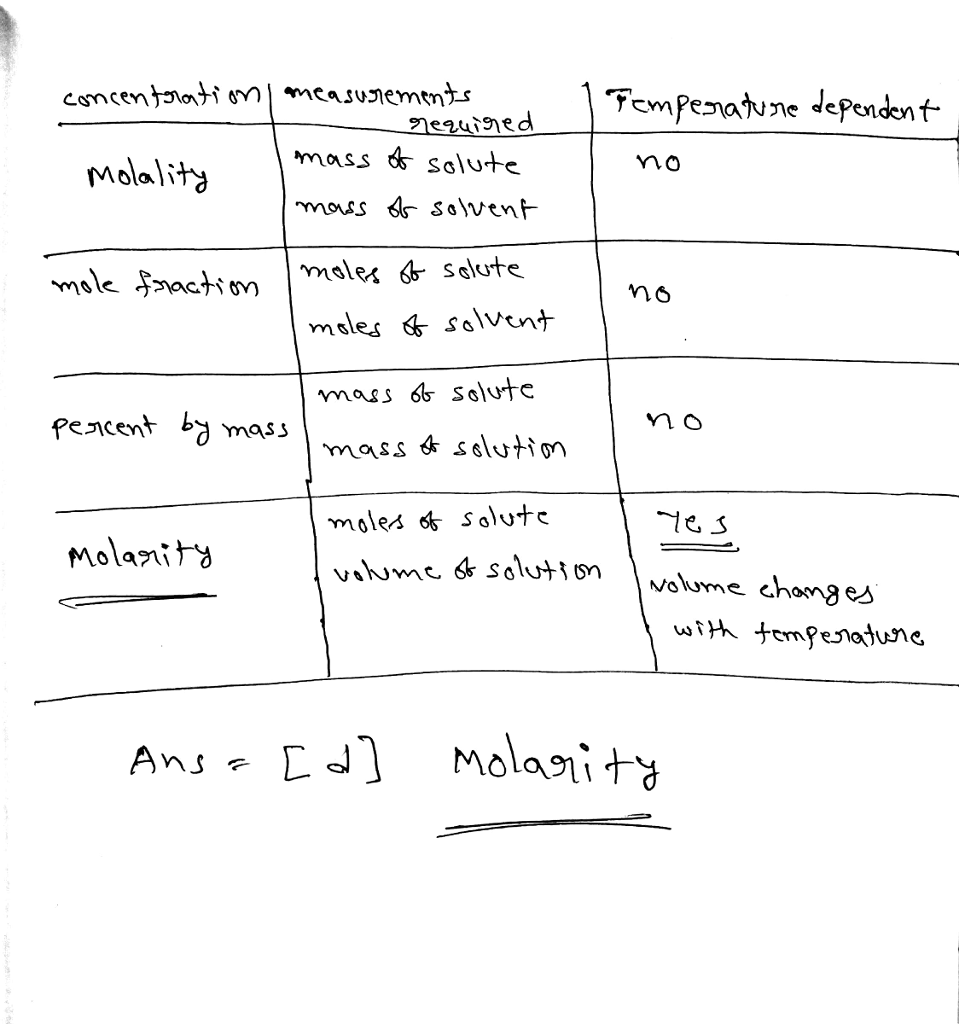# Homework Solution: Which of the following is temperature dependent for a solution? a) Molality b)…

Which of the following is temperature dependent for a solution? a) Molality b) Mole fraction c) Percentage by mass d) Molarity